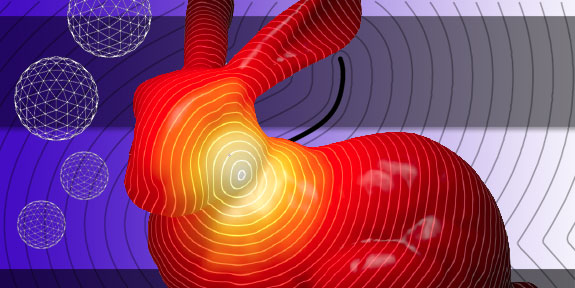# Research > Graphics & Geometry## Overview

Our research on graphics and discrete differential modeling is based around the insight that numerical simulation and modeling on a computer require discrete versions of the continuous mathematical models describing these systems. For these computations to be truly predictive, reliable, and efficient the underlying continuous structures must be transferred to the discrete systems. The goal of the research at Caltech in this area is to identify the relevant mathematical and computer science tools to lay the foundation for the rational construction of such discrete differential modeling tools which preserve the relevant structures. The study of geometry in a broad sense forms the core of this area but it also draws considerably on fields ranging from algebraic topology to computational geometry, graph theory, combinatorics, applied mathematics, and computer science. Application areas include computer graphics, variational mechanics, and biological systems.

## Faculty

Related research groups & Centers > Graphics Group, Multires Modeling Group, Applied Geometry Lab

## Related Courses

ACM/CMS 104. Linear Algebra and Applied Operator Theory.
ACM 106 ab. Introductory Methods of Computational Mathematics.
ACM/CS 114 ab. Parallel Algorithms for Scientific Applications.
EE/CNS/CS 148 ab. Selected Topics in Computational Vision.
CS/CNS 171. Introduction to Computer Graphics Laboratory.
CS/CNS 174. Computer Graphics Projects.
CS 176. Introduction to Computer Graphics Research.
CS 177. Discrete Differential Geometry: Theory and Applications.
CS 179. GPU Programming.
CS 274 abc. Topics in Computer Graphics.